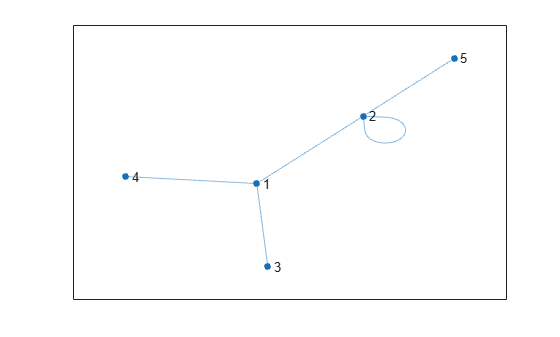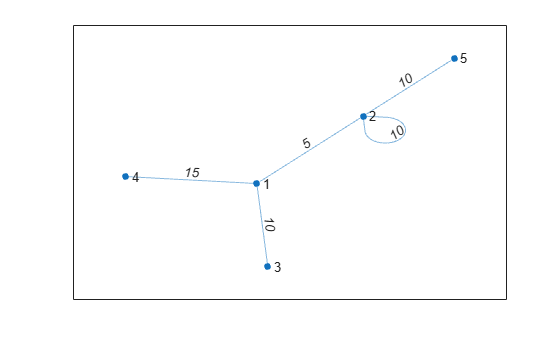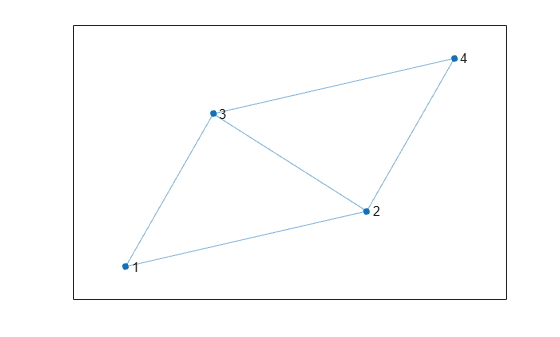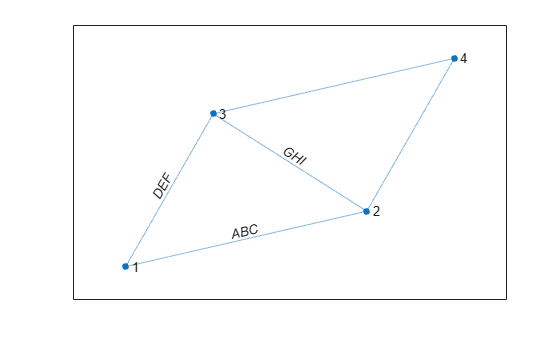Documentation

### This is machine translation

Mouseover text to see original. Click the button below to return to the English version of the page.

Note: This page has been translated by MathWorks. Click here to see
To view all translated materials including this page, select Country from the country navigator on the bottom of this page.

# labeledge

Label graph edges

## Syntax

``labeledge(H,s,t,Labels)``
``labeledge(H,idx,Labels)``

## Description

example

````labeledge(H,s,t,Labels)` labels the edges specified by `(s,t)` node pairs with the character vectors or strings contained in `Labels`. The lengths of `s`, `t`, and `Labels` must be equal, or `Labels` can be scalar. If there are multiple edges between `s` and `t`, then the same label is applied to all of them.```

example

````labeledge(H,idx,Labels)` specifies the edges to label using logical or numeric edge indices.```

## Examples

collapse all

Create and plot a graph.

```s = [1 1 1 2 2]; t = [2 3 4 2 5]; G = graph(s,t); h = plot(G);```Label the edge weights using `labeledge`.

```weights = [5 10 15 10 10]; labeledge(h,1:numedges(G),weights)```Create and plot a graph.

```s = [1 1 2 2 3]; t = [2 3 3 4 4]; G = graph(s,t); h = plot(G);```Add text labels to three of the five graph edges.

```labelText = {'ABC' 'DEF' 'GHI'}; labeledge(h,[1 1 2],[2 3 3],labelText)```## Input Arguments

collapse all

Input graph plot, specified as a `GraphPlot` object. Use the `graph` or `digraph` functions to create a graph, and then use `plot` with an output argument to return a `GraphPlot` object.

Example: `H = plot(G)`

Node pairs, specified as separate arguments of node indices or node names. Similarly located elements in `s` and `t` specify the source and target nodes for edges in the graph.

This table shows the different ways to refer to one or more nodes either by their numeric node indices or by their node names.

FormSingle NodeMultiple Nodes
Node index

Scalar

Example: `1`

Vector

Example: `[1 2 3]`

Node name

Character vector

Example: `'A'`

Cell array of character vectors

Example: `{'A' 'B' 'C'}`

String scalar

Example: `"A"`

String array

Example: `["A" "B" "C"]`

Example: ```labeledge(H,[1 2 2],[2 3 4],{'label1' 'label2' 'label3'})```

Example: ```labeledge(H,{'a' 'b' 'b'},{'b' 'c' 'd'},{'label1' 'label2' 'label3'})```

Edge indices, specified as a scalar, vector of positive integers, or logical vector.

• A vector of positive integers specifies edge indices for a graph `G` corresponding to rows in the table `G.Edges`, such that `G.Edges(idx,:)` returns the end nodes of the edge. In this form, the maximum value for `idx` is `numedges(G)`, and the number of selected nodes is equal to the number of indices in `idx`.

• A logical vector specifies whether each edge is labeled. In this form, the length of the logical vector must be `numedges(G)`, and the number of selected nodes is equal to the number of nonzero indices in `idx`.

Example: ```labeledge(H,[1 3 5 7],{'label1' 'label2' 'label3' 'label4'})```

Edge labels, specified as a character vector, string scalar, cell array of character vectors, or string array.

• If `Labels` is a character vector or string scalar, then `labeledge` uses that label for each specified edge.

• If `Labels` is a cell array or string array, then it must contain a label for each specified edge.

Data Types: `char` | `cell` | `string`

Download ebook